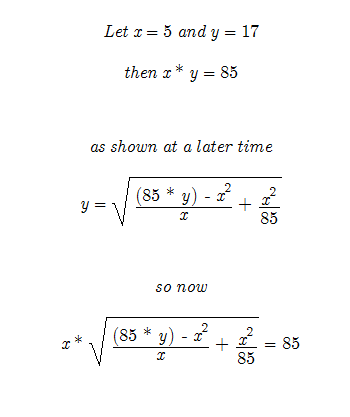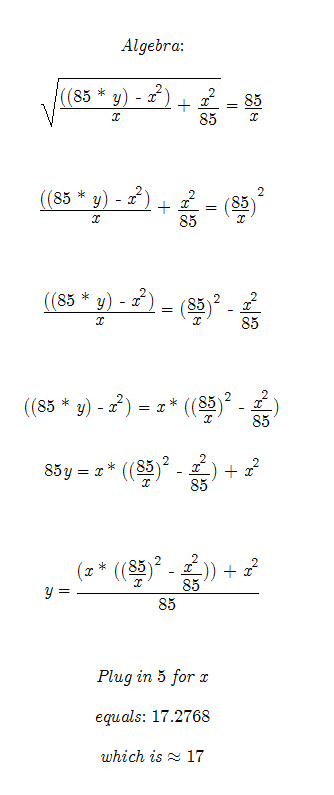Again I will have to explain where the equation was derived in a future post. For now compare to Here!

All that is needed is another equation to substitute the x values of y. The trouble is it is a fourth order equation and there is much mess with the substitution equation. Solving for x is the ultimate goal. However this equation can still prove useful. It may be more useful as I show it derived.

But until then, this is where the equation stands.